# Axis and Title Modifications in ggplot2

This post will provide explanation on how to customize the axis and title of a plot that utilizes ggplot2. We will use the “Computer” dataset from the “Ecdat” package looking specifically at the difference in price of computers based on the inclusion of a cd-rom. Below is some code needed to be prepared for the examples along with a printout of our initial boxplot.

``library(ggplot2);library(grid);library("Ecdat")``
``````data("Computers")
theBoxplot<-ggplot(Computers,aes(cd, price, fill=cd))+geom_boxplot()
theBoxplot``````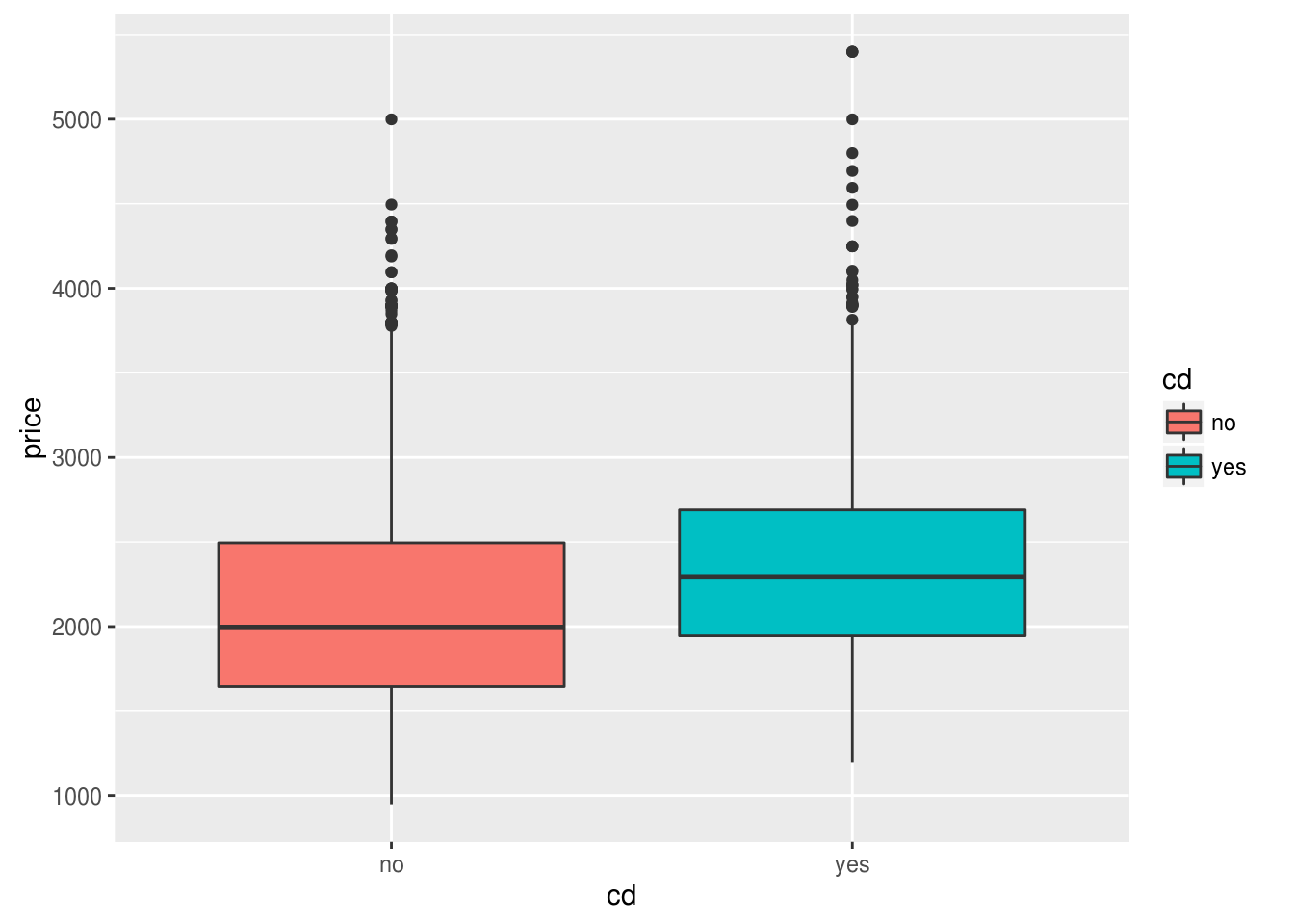In the example below, we change the color of the tick marks to purple and we bold them. This all involves the use of the “axis.text” argument in the “theme” function.

``theBoxplot + theme(axis.text=element_text(color="purple",face="bold"))``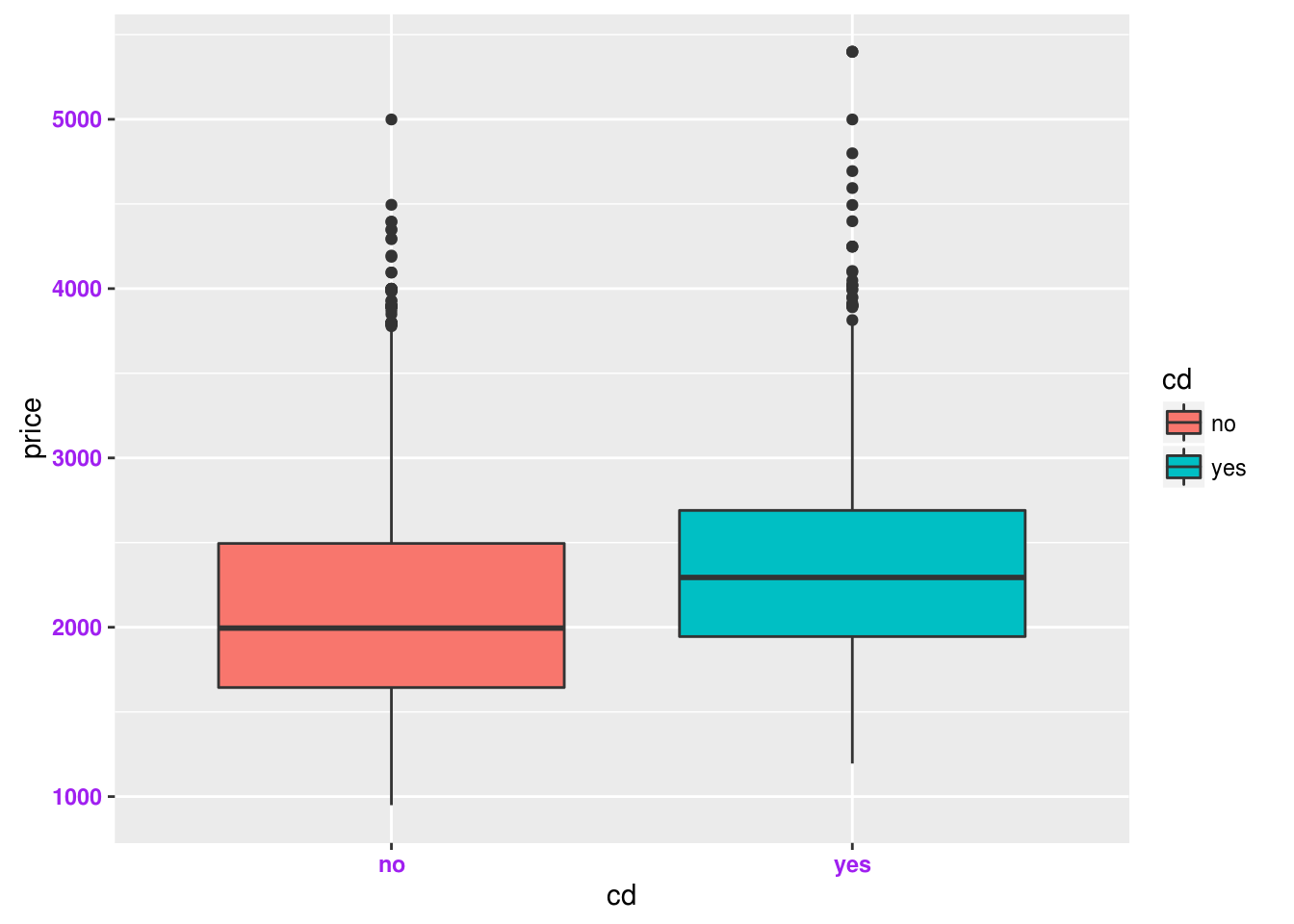In the example below, the y label “price” is rotated 90 degrees to be in line with text. This is accomplished using the “axis.title.y” argument along with additional code.

``theBoxplot+theme(axis.title.y=element_text(size=rel(1.5),angle = 0))``Below is an example that includes a title with a change to the default size and color

``theBoxplot+labs(title="The Example")+theme(plot.title=element_text(size=rel(1.5),color="orange"))``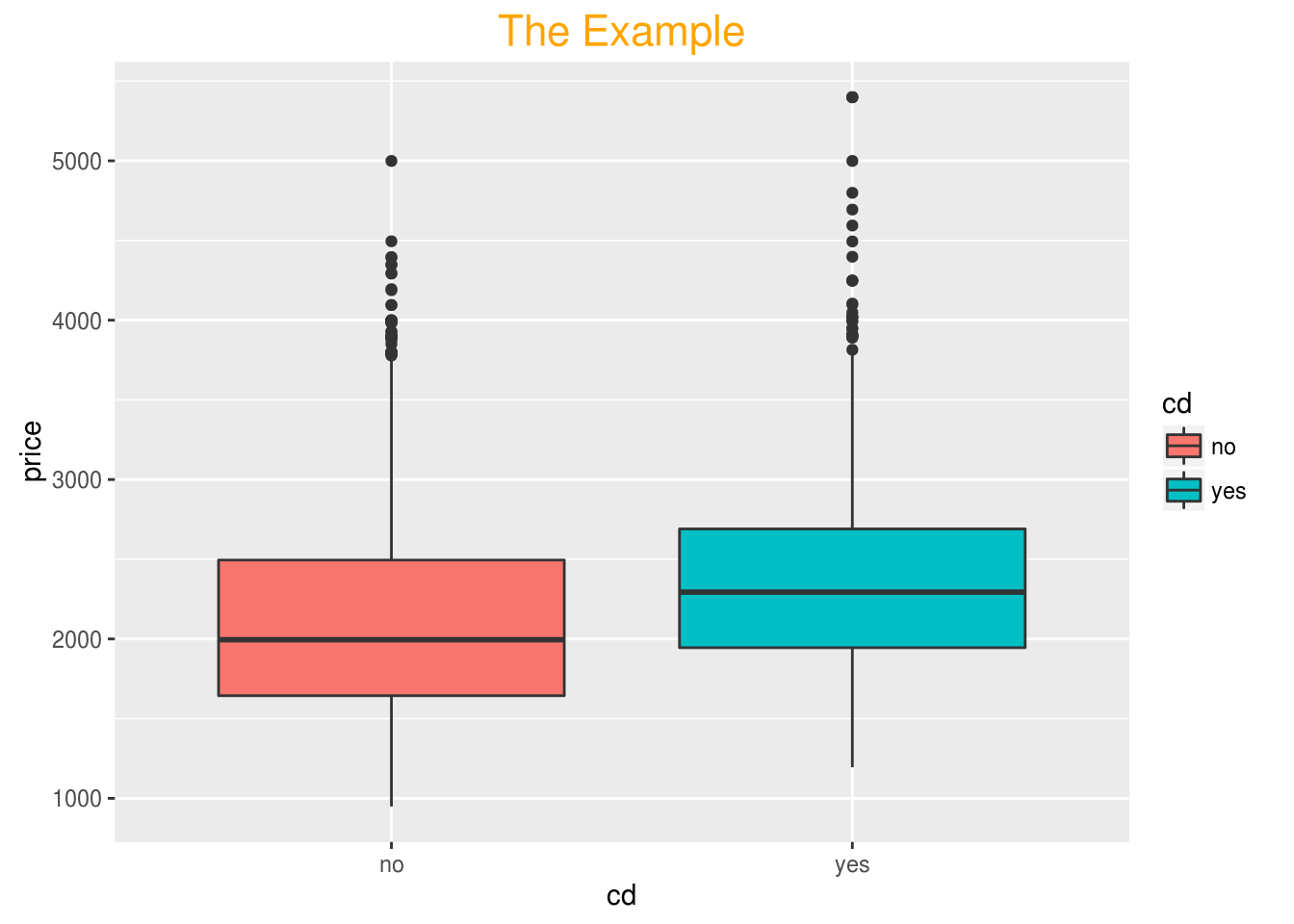You can also remove the axis label. IN the example below, we remove the x axis along with its tick marks.

``theBoxplot+theme(axis.text.x=element_blank(),axis.ticks.x=element_blank(),axis.title.x=element_blank())``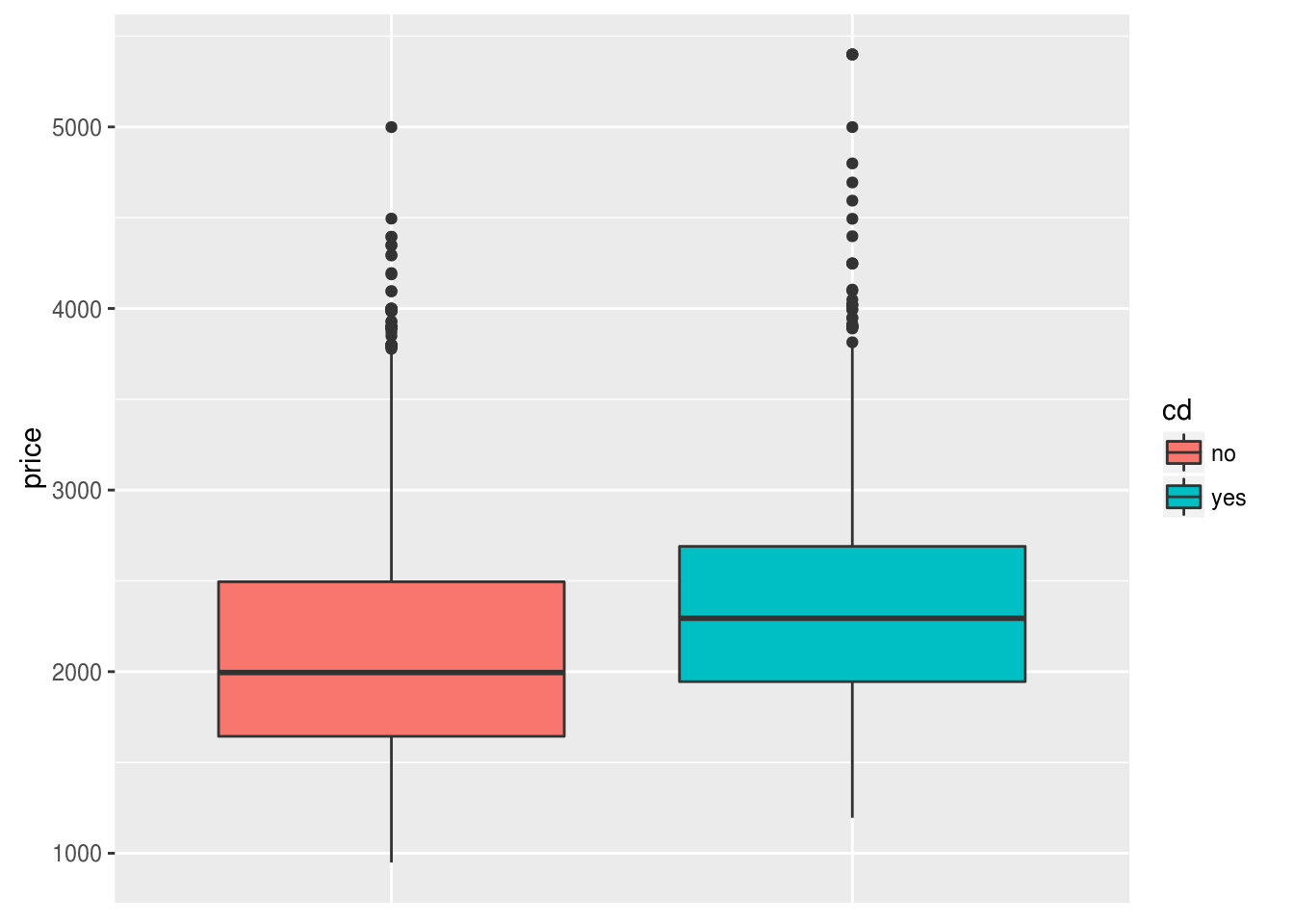It is also possible to modify the plot background axis as well. In the example below, we change the background color to blue, the color of the lines to green, and yellow.

This is not an attractive plot but it does provide an example of the various options available in ggplot2

``theBoxplot+theme(panel.background=element_rect(fill="blue"), panel.grid.major=element_line(color="green", size = 3),panel.grid.minor=element_line(color="yellow",linetype="solid",size=2))``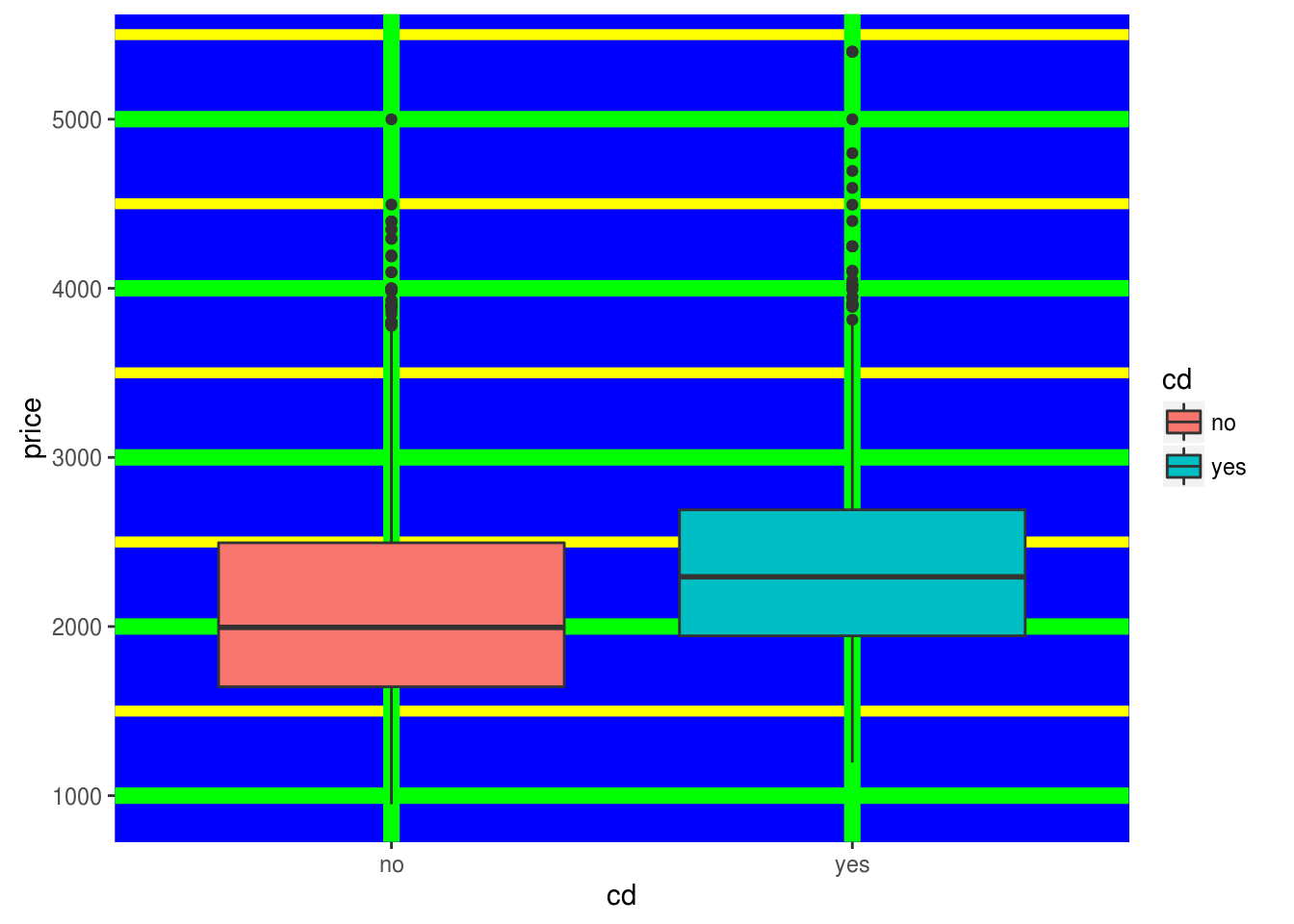All of the tricks we have discussed so far can also apply when faceting data. Below we make a scatterplot using the same background as before but comparing trend and price.

``````theScatter<-ggplot(Computers,aes(trend, price, color=cd))+geom_point()
theScatter1<-theScatter+facet_grid(.~cd)+theme(panel.background=element_rect(fill="blue"), panel.grid.major=element_line(color="green", size = 3),panel.grid.minor=element_line(color="yellow",linetype="solid",size=2))
theScatter1``````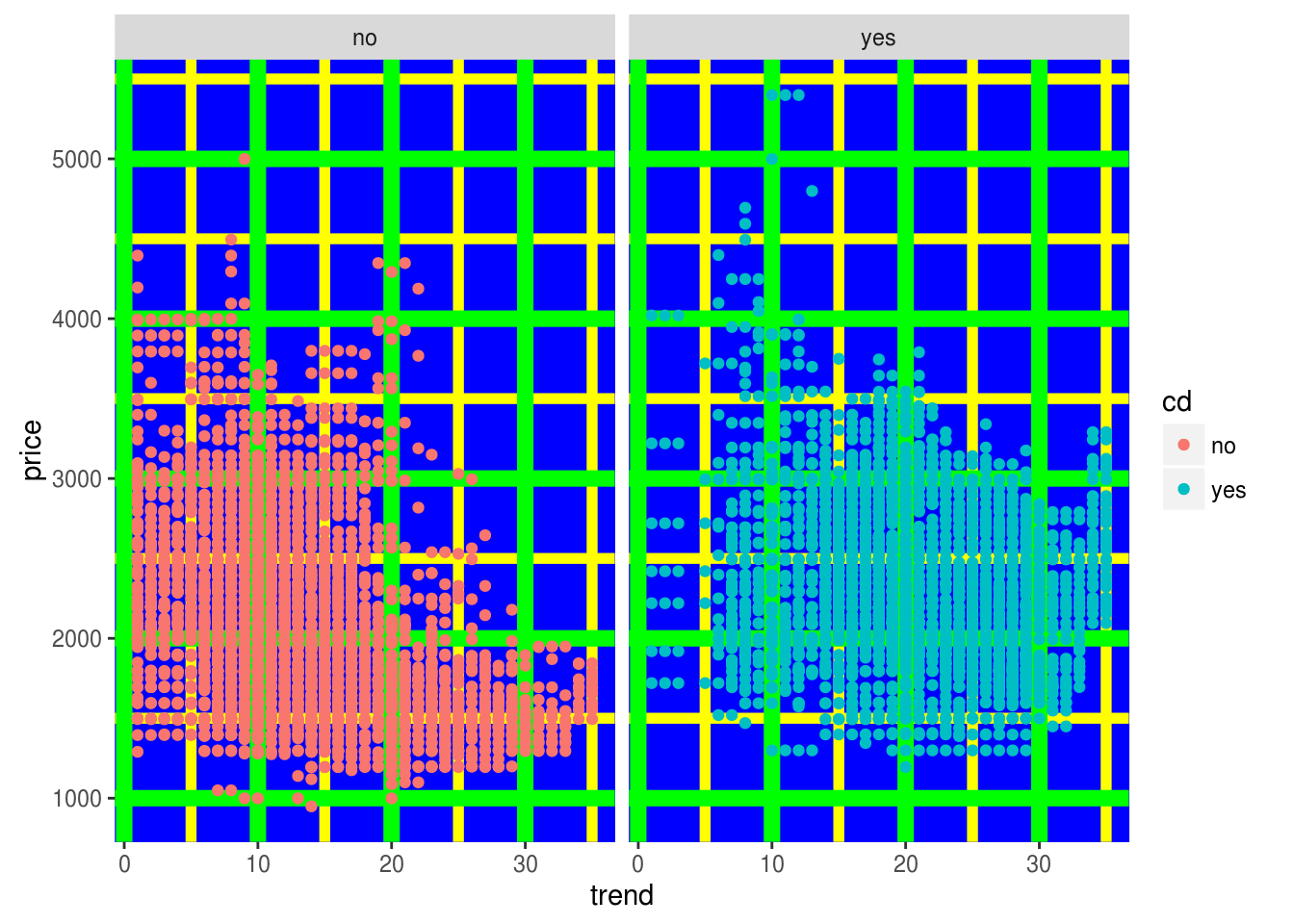Right now the plots are too close to each other. We can account for this by modifying the panel margins.

``theScatter1 +theme(panel.margin=unit(2,"cm"))``Conclusion

These examples provide further evidence of the endless variety that is available when using ggplot2. Whatever are your purposes, it is highly probably that ggplot2 has some sort of a data visualization answer.